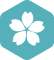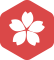### Python中inplace=True的理解GitHub 绑定GitHub第三方账户获取2015年4月 扩充话题大版内专家分月排行榜第三2014年4月 扩充话题大版内专家分月排行榜第二daixiangcnGitHub 绑定GitHub第三方账户获取
python pandas inplace 参数理解

pandas inplace 参数在很多函数中都会有，它的作用是：是否在原对象基础上进行修改 ​ inplace = True：不创建新的对象，直接对原始对象进行修改； ​ inplace = False：对数据进行修改，创建并返回新的对象承载...

pandas中inplace_Python_Pandas中inplace=Ture 的理解

inplace参数的理解：修改一个对象时：inplace=True：不创建新的对象，直接对原始对象进行修改；inplace=False：对数据进行修改，创建并返回新的对象承载其修改结果。student['Age']=np.arange(0,len(student))#增加...

loctionplace.reset_index(drop=True,inplace=True)

loctionplace.reset_index(drop=True,inplace=True)@TOC

Pandas inplace=True 可以节约内存么？

inplace=True 参数不会创建新的对象，只修改原对象，所以可以实现节约内存的目的，我的理解正确么？

pandas 如何修改列名 rename方法【必须增加inplace=True才会生效】

""" perf.columns的结果为： Index(['Unnamed: 0', 'algo_volatility', 'algorithm_period_return', 'alpha', 'benchmark_period_return', 'benchmark_volatility', 'beta', 'capital_used', 'en...

pandas.DataFrame.drop_duplicates后面inplace=Trueinplace=False的区别

t.drop_duplicates(inplace=True) 则，对t重复将被去除。   drop_duplicates(inplace=False)将不改变原来的dataFrame，而将结果生成在一个新的dataFrame。 如： s = t.drop_dup...

PyTorchnn.ReLU(inplace=True)中inplace的作用

sort is deprecated, use sort_values(inplace=True) for INPLACE sorting

PyTorchnn.ReLU() 和 nn.ReLU(inplace=True)区别

nn.ReLU() 默认的 inplace=False, 这个inplace意思：是否将计算得到的值直接覆盖之前的值 如果，inplace=True，那么就是会对原变量覆盖，没有通过中间变量，直接覆盖原变量的值 比如： x=x+1 如果，inplace=False...

Python中inplace、subset参数的意义

Python使用，很多函数都会有inplace这个参数。 df.drop(['CLOSE'],axis=1,inplace=True) Inplace = True意思就是df.drop()函数执行完之后，直接赋值给df这个对象，如果Inplace = False则df.drop()只是执行了...

Python drop方法删除列之inplace参数

drop方法有一个可选参数inplace，表明可对原数组作出修改并返回一个新数组。不管参数默认为False还是设置为True，原数组的内存值是不会改变的，区别在于原数组的内容是否直接被修改。默认为False,表明原数组内容并不...

python inplace

python inplace用法 出自链接 https://blog.csdn.net/songyunli1111/article/details/82937954 pandas inplace 参数在很多函数中都会有，它的作用是：是否在原对象基础上进行修改 ​ inplace = True：不创建新的...

pandas笔记2---reset_index函数drop与inplace参数的理解

df.rename(columns=lambda x: x.strip().lower().replace(' ','_'),inplace=True)里的参数问题

df_18.rename(columns=lambda x: x.strip().lower().replace(' ','_'),inplace=True) 这是一个在数据集中用下划线和小写标签代替空格的代码 我想问的是为什么coulumns后面我没输入列名，只是一个函数，而且这个...

python 使用pandas的dropna方法过滤缺失数据

DataFrame.dropna(axis=0, how=‘any’, thresh=None, subset=None, inplace=False) 参数：axis : 0为删除行，1为删除列 import pandas as pd import numpy as np df=pd.DataFrame(np.random.randn(4,5),c...

Python-Pandas数据排序，sort_index()与sort_values()

sort_index(axis=0, level=None, ascending=True, inplace=False, kind=‘quicksort’, na_position=‘last’, sort_remaining=True, by=None) axis：0按照行名排序；1按照列名排序 level：默认N...

python进行数据处理——pandas的drop函数

fillna()函数填充报错SettingWithCopyWarning

X['Age'].fillna(X['Age'].mean(), inplace=True)运行后会出现 Warning (from warnings module): File "D:\Python\Python27\lib\site-packages\pandas\core\generic.py", line 6130 self._update_inplace(new_...

Learning Pandas - fillna()填充缺失数据

fillna()函数详解

inplace参数的取值：True、False True：直接修改原对象 False：创建一个副本，修改副本，原对象不变（缺省默认） method参数的取值 ： {‘pad’, ‘ffill’,‘backfill’, ‘bfill’, None}, def...

[Python] Pandas之sort_values isin使用技巧

1.在pandas的DataFrame，我们经常需要根据某属性来选取指定条件的行，这时isin方法就特别有效。 import pandas as pd df = pd.DataFrame([[1,2,3],[1,3,4],[2,4,3]],index = ['one','two','three'],columns = ['A'...

ReLU之参数 inplace=True

pandas 对axis=0,axis=1的理解，对应pandasdrop的用法

Stackoverflow.com是程序员的好去处，本公众号将以pandas为主题，开始一个系列，争取做到每周一篇，翻译并帮助pandas学习者一起理解一些有代表性的案例。...python中的axis究竟是如何定义的呢？他们究...

Python进行数据处理之Pandas的drop函数

pandas dataframe 选择列后 fillna() 使用inplace参数

pandas dataframe 选择列后 fillna() 使用inplace参数，不改变源数据。 df4=pd.DataFrame(data=np.arange(0,20).reshape(5,4),columns=['c1','c2','c3','c4'],index=range(0,5)) df4.loc[2,'c2']=np.nan df4 df4...

python中nan值判断与处理

2020美赛O奖论文.zip

2020年美赛C题O奖论文（含6篇）

2020年美赛C题O奖论文（含6篇）